﻿ Point Group Flowchart

## Crystal Class Determination Flowchart

Every crystal can be classified into one of 32 point groups. These groups are a collection of rotations and rotoinversions that leave the crystal looking the same as before their application.

This page is an interactive flowchart to determine the point group of an object depending on it's symmetries. The flowchart is taken from Breneman 1987 & Casas 1992.

After clicking start there will be a series of questions to determine the type of point group your crystal posseses. See the legend at the bottom of the page for more information about each operation and how they are depicted.
The end result is one of the 32 point groups given by it's class name, short Herman-Maugin notation and a link to the table of properties of the classes.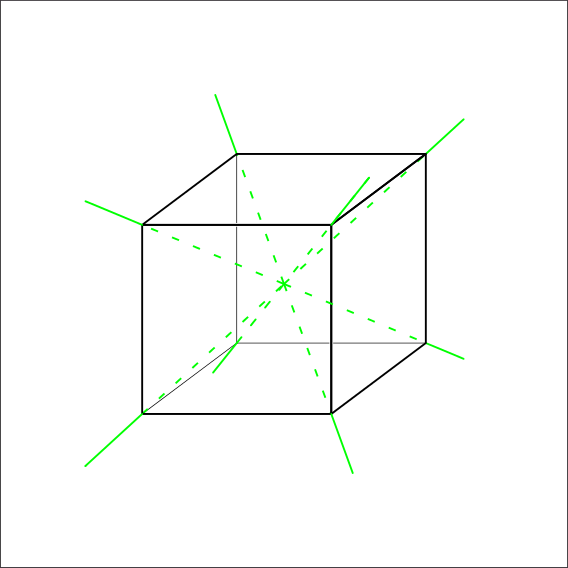Does rotation around all four body diagonals have 3-fold symmetry?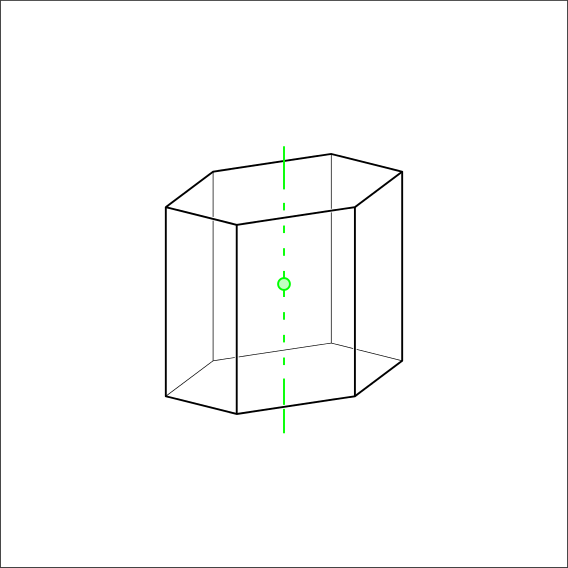Is there a 6-fold symmetry or 6 Rotoinversion?Is there a 4-fold symmetry or 4 Rotoinversion?Is there a 3-fold symmetry or 3 Rotoinversion?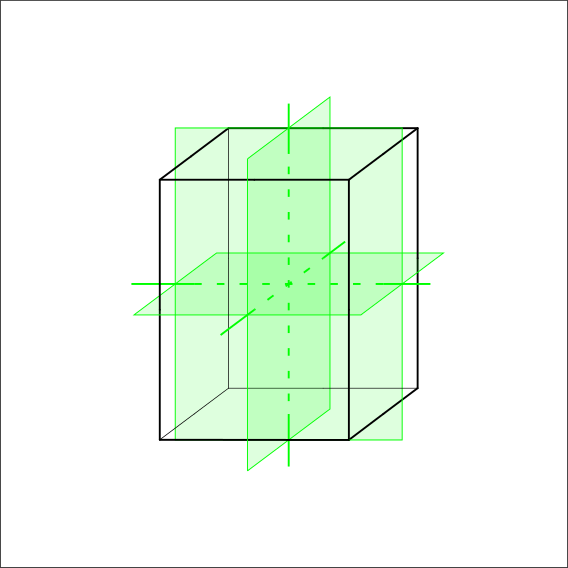Does the object have three 2-fold symmetry axis and/or mirror planes that are perpendicular to each other?Is there a 2-fold symmetry axis and/or mirror plane?Is there a 1-fold symmetry or 1 rotoinversion?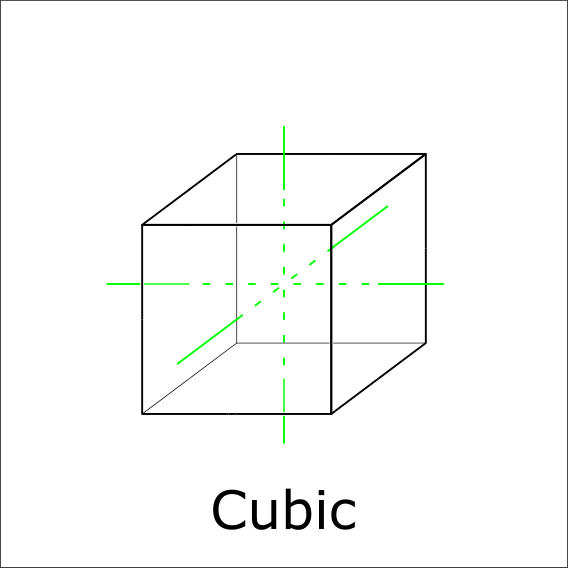Are there three 4-fold rotations through the center?Are there three 4-fold rotoinversions through the center?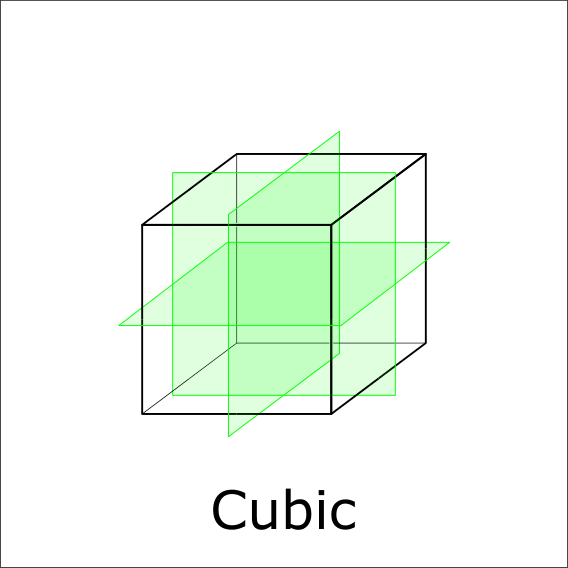Is there a mirror plane perpendicular to the 4-fold axis?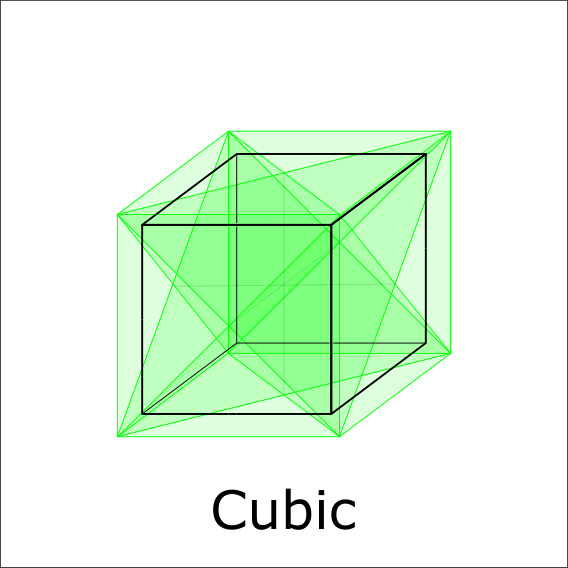Is there a mirrorplane perpendicular to a 4-fold axis?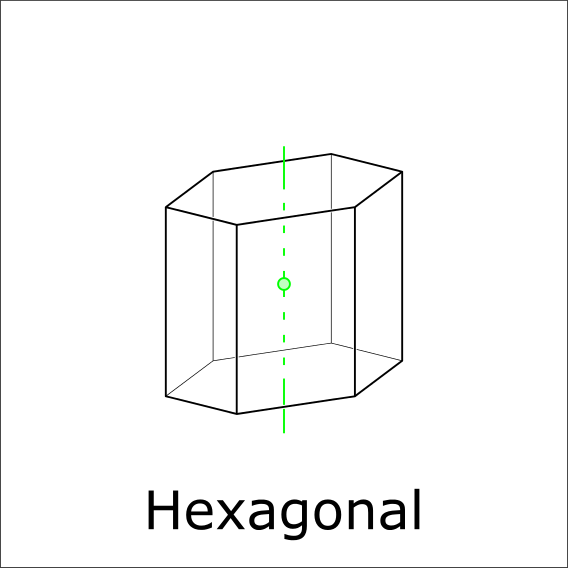Is there a 6-fold rotoinversion?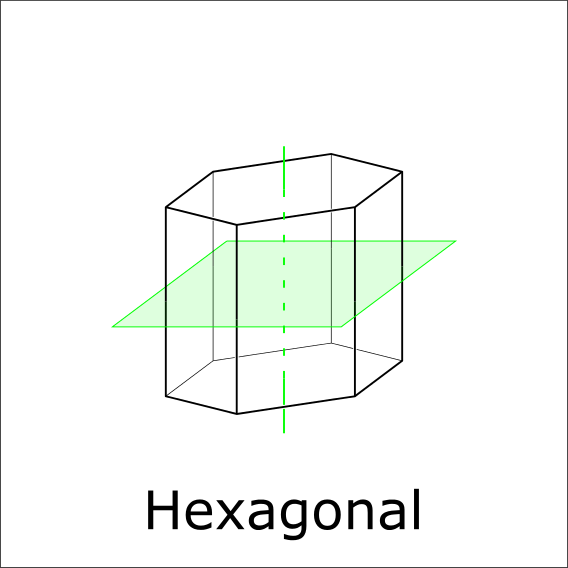Is there a mirror plane perpendicular to the 6-fold rotational axis?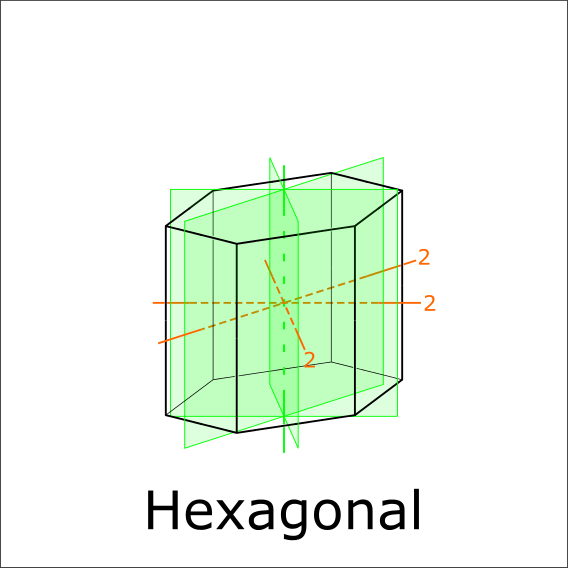Are there mirror planes or 2-fold symmetries?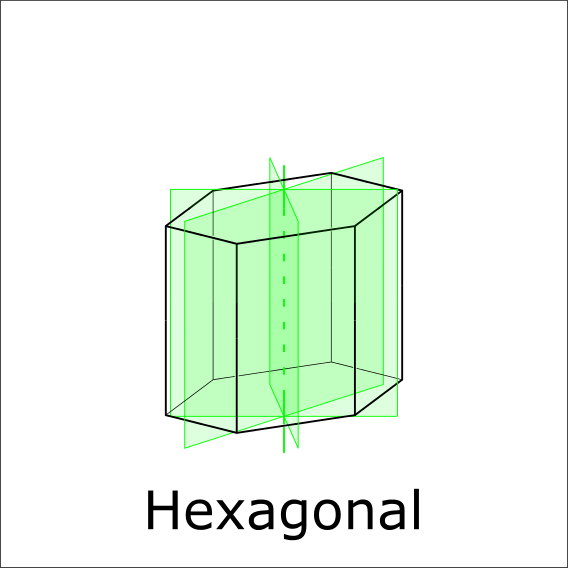Mirror planes or 180° rotations?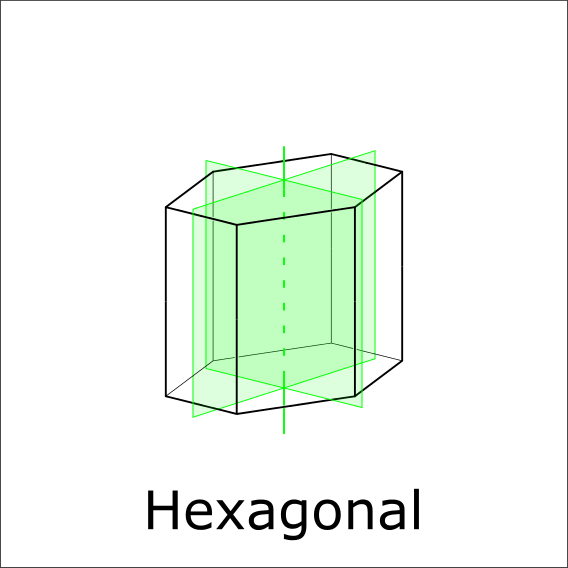Additional mirror planes parallel to the 6-fold axis?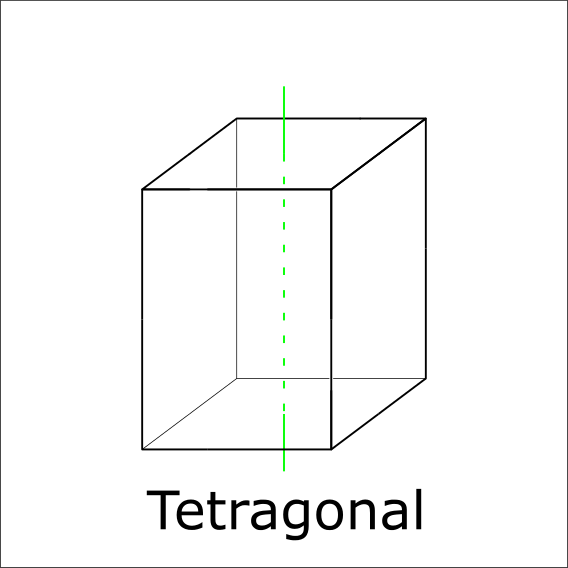Is there a 4-fold symmetry axis?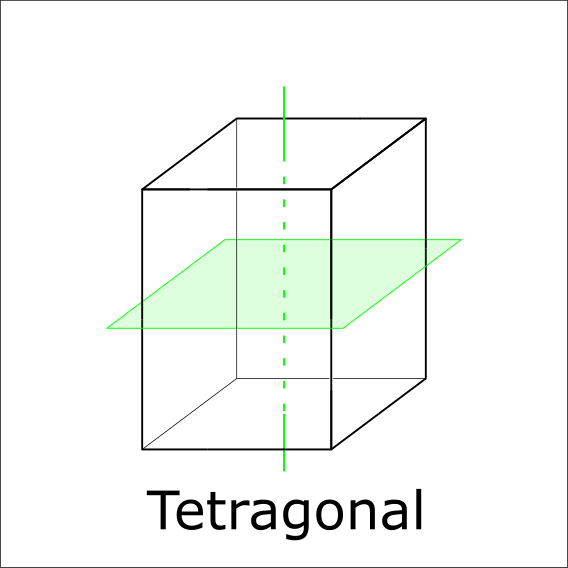Is there a mirror plane perpendicular to the 4-fold axis?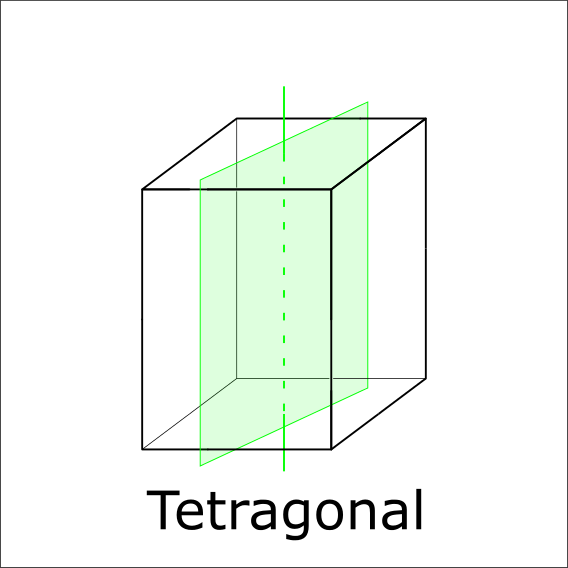Is there a mirror plane paralell to the 4-fold axis?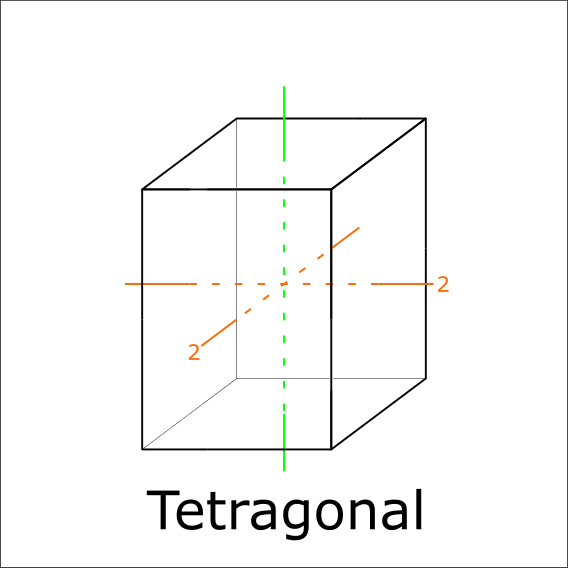Are there two 2-fold rotational axis perpendicular to the 4-fold axis?Any additional mirror planes parallel to the 4-fold axis?Is there 3 rotoinversion?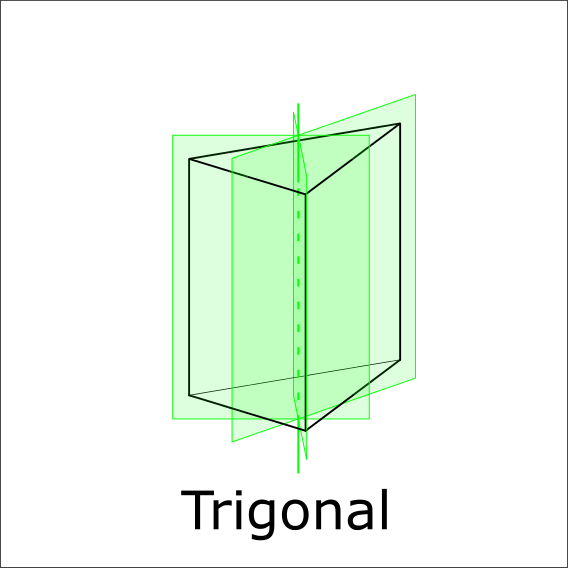Are there three mirror planes paralell to the 3-fold axis?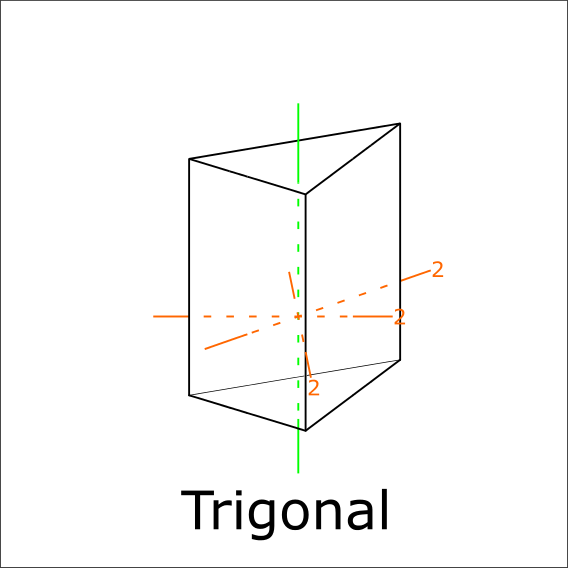Are there three mirror planes perpendicular to the 3-fold axis?Are there three mirror planes paralell to the 3 rotoinversion axis?Are there three perpendicular mirror planes?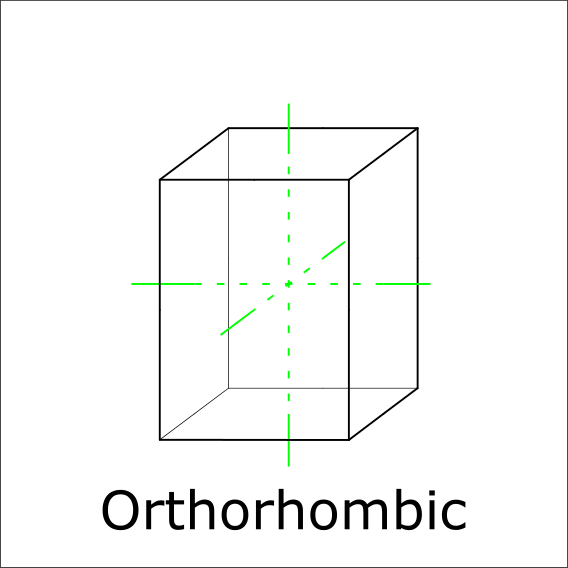Are there three perpendicular 2-fold axis?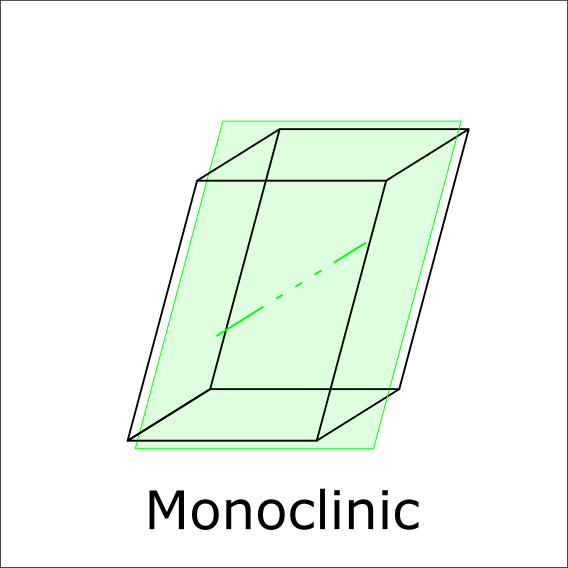Is there a perpendicular mirrorplane to the 2-fold axis?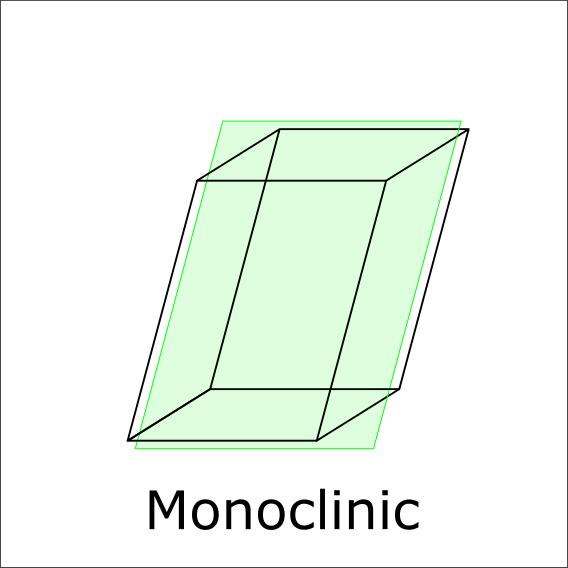Is there a mirror plane?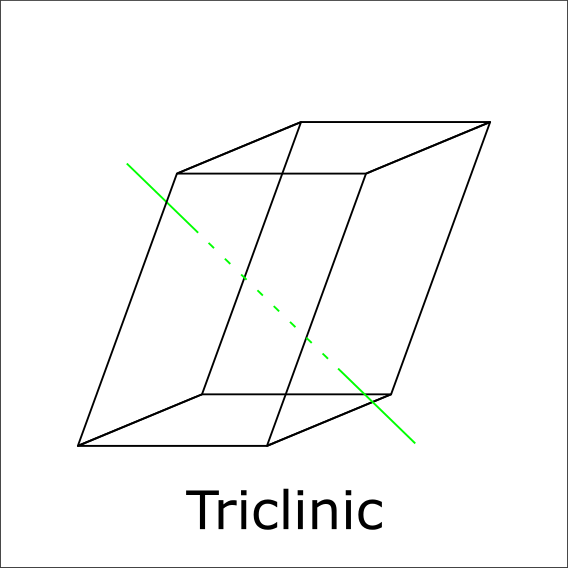Is there a 1-fold symmetry?Are there mirror planes parallel to the 6 rotoinversion axis which are 120° apart?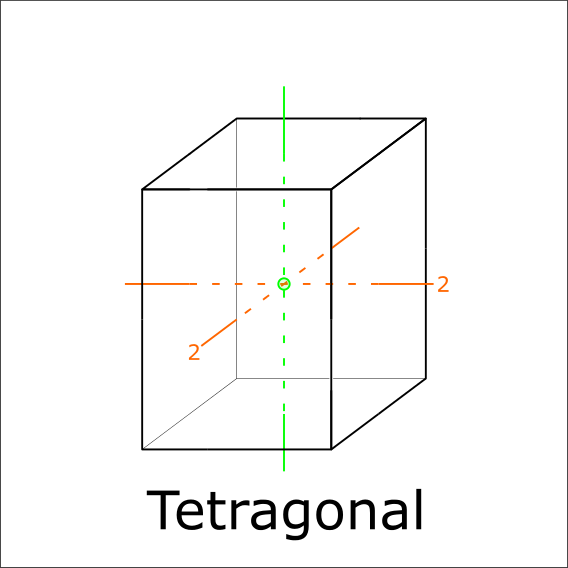Are there two 2-fold rotations perpendicular to the 4-rotoinversion axis?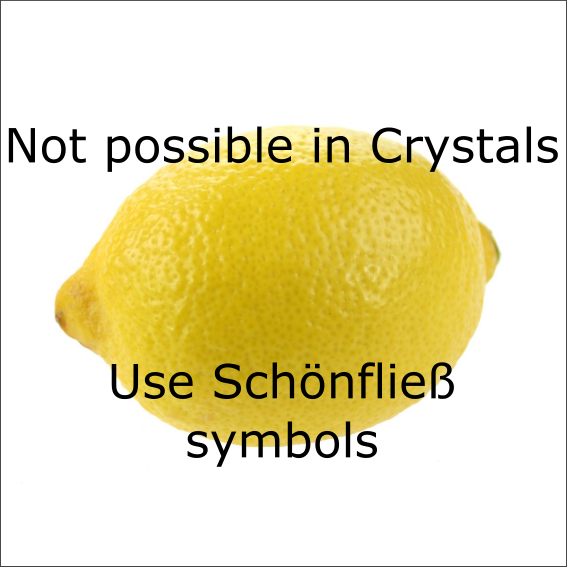#### Legend

• n-fold symmetry (1,2,3,4,6): Rotation around an axis looks the same as the original for every 360/n° turn.
Represented by green lines
• Rotoinversion (1,2,3,4,6): Same as n-fold symmetry, but after turning the object is mirrored along a plane perpendicular to the rotation axis.
Rotoinversions are represented by lines with a circle at the inversion center
• Mirror plane (m = 2):
Represented by light green planes.